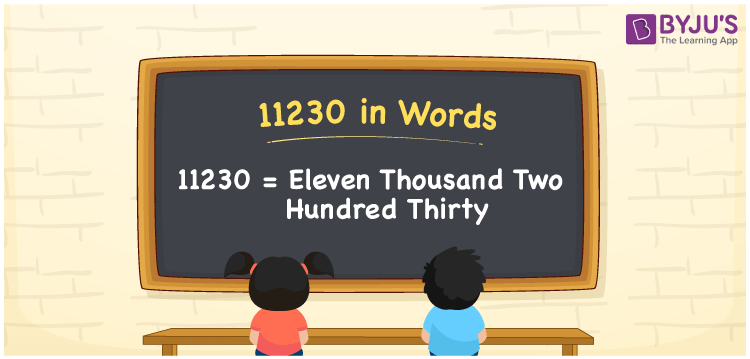# 11230 in words

11230 in words is written as Eleven Thousand Two Hundred and Thirty. In 11230, the first 1 has a place value of ten thousand, second 1 is in the place value of thousand, 2 is in the place value of hundred and 3 is in the place value of ten. The article on Place Value gives more information. The number 11230 is used in expressions that relate to money, distance, Social media views, and many more. For example, “The cost of that gold ring is Eleven Thousand Two Hundred and Thirty rupees.”

 11230 in words Eleven Thousand Two Hundred and Thirty Eleven Thousand Two Hundred and Thirty in Numbers 11230

## 11230 in English Words## How to Write 11230 in Words?

We can convert 11230 to words using a place value chart. The number 11230 has 5 digits, so let’s make a chart that shows the place value up to 5 digits.

 Ten thousand Thousands Hundreds Tens Ones 1 1 2 3 0

Thus, we can write the expanded form as:

1 × Ten thousand + 1 × Thousand + 2 × Hundred + 3 × Ten + 0 × One

= 1 × 10000 + 1 × 1000 + 2 × 100 + 3 × 10 + 0 × 1

= 11230.

= Eleven Thousand Two Hundred and Thirty.

11230 is the natural number that is succeeded by 11229 and preceded by 11231.

11230 in words – Eleven Thousand Two Hundred and Thirty.

Is 11230 an odd number? – No.

Is 11230 an even number? – Yes.

Is 11230 a perfect square number? – No.

Is 11230 a perfect cube number? – No.

Is 11230 a prime number? – No.

Is 11230 a composite number? – Yes.

## Solved Example

1. Write the number 11230 in expanded form

Solution: 1 x 10000 + 1 x 1000 + 2 x 100 + 3 x 10 + 0 x 1

We can write 11230 = 10000 + 1000 + 200 + 30 + 0

= 1 x 10000 + 1 x 1000 + 2 x 100 + 3 x 10 + 0 x 1.

## Frequently Asked Questions on 11230 in words

Q1

### How to write the number 11230 in words?

11230 in words is written as Eleven Thousand Two Hundred and Thirty.
Q2

### Is 11230 a prime number?

No. 11230 is not a prime number.
Q3

### Is 11230 divisible by 10?

Yes. 11230 is divisible by 10.